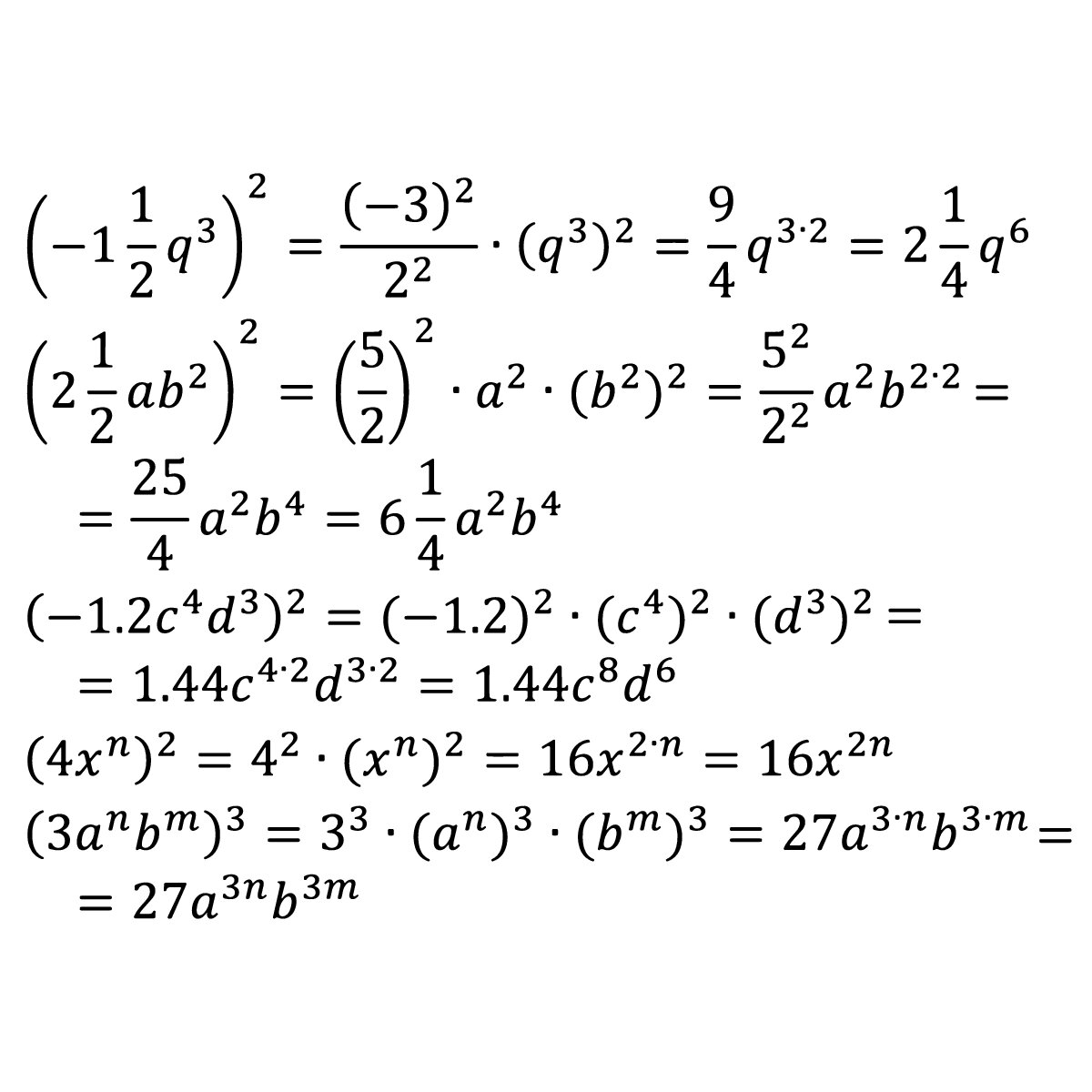# Hey ALGEBRA Students, Don’t Let Negative Exponents Get You Down!

A parent or student that’s unfamiliar with basic algebra may be scratching their head right about now. What is a negative exponent? No, it’s not an exponent that’s grumpy or sad, nor is it a negative number. Pictorially, it’s an exponent with a negative sign in front of it.It’s August, and time to go back to school.

For many basic algebra students in pre-algebra and basic algebra in Huntington Beach,

this will be their first time being introduced to negative exponents.  Understanding what a negative exponent is, where it’s used in real life, and how to solve negative exponents can be tricky,

but with a home algebra tutor, they’re much easier!

A parent or student that’s unfamiliar with basic algebra may be scratching their head right about now.  What is a negative exponent?  No, it’s not an exponent that’s grumpy or sad, nor is it a negative number. Pictorially, it’s an exponent with a negative sign in front of it.

A home algebra tutor in Huntington Beach can explain that the negative exponent

of a number is equal to the reciprocal of the positive exponent of that number.  For example:

This is true for any number.  In algebra, the “m” above can be an integer, fraction, decimal, irrational, or even imaginary number.  As long as there’s a negative sign in front of it, it’s a negative exponent.

In basic algebra, where are negative exponents used?

Negative exponents are typically used to show numbers much smaller than one.  In scientific notation, while 10^positive integer means that the number is greater than one to a power of ten, 10^negative integer means that the number is smaller than one by a power of ten.  You may see them in Chemistry or Physics when talking about very small sizes, speeds, or distances.

Here are a couple of other basic algebra examples of solving a negative exponent.  Remember, to solve, you can NOT have any negative exponents in your final answer:

The other important trick to remember when solving these challenging algebra problems is that a negative exponent is NOT the same thing as a negative number.  That negative sign can be tricky,

but an outstanding home algebra tutor

can keep reminding and drilling the idea that negative exponents and negative numbers are not the same things.  Negative exponents mean the reciprocal of the original number.

Did you or your child struggle in math last year?  Get yourself on the right track by hiring a weekly home algebra tutor at the start of the school year! A Plus In Home algebra tutors offers tutoring for all subjects including basic algebra in Huntington Beach, Newport Beach, Sunset Beach, and throughout Orange County.

A great home algebra tutor in Huntington Beach can help increase your comprehension and your test scores.